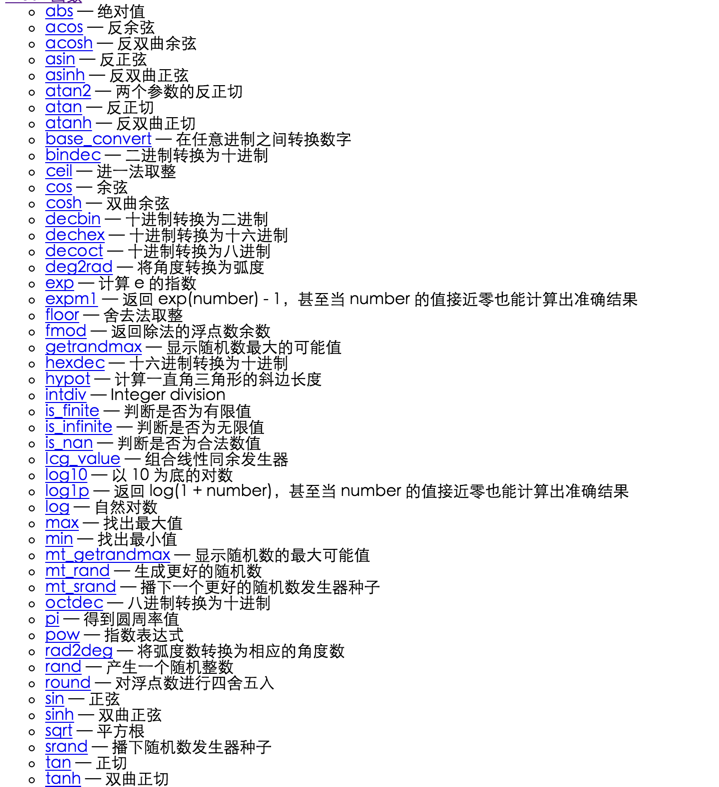• 注册

# php 数学常用函数abs()求绝对值\$abs = abs(-4.2); //4.2数字绝对值数字
ceil()进一法取整echo ceil(9.999); // 10浮点数进一取整
floor()舍去法取整echo floor(9.999); // 9浮点数直接舍去小数部分
fmod()浮点数取余"\$x = 5.7;\$y = 1.3;\$r = fmod(\$x, \$y);// \$r equals 0.5, because 4 *  1.3 + 0.5 = 5.7    "两个浮点数,x>y浮点余数
pow()返回数的n次方echo pow(-1, 20); // 1基础数 n次方乘方值
round()浮点数四舍五入echo round(1.95583, 2);// 1.96一个数值保留小数点后多少位,默认为0 舍入后的结果
sqrt()求平方根echo sqrt(9); //3被开方的数平方根
max()求最大值"echo max(1, 3, 5, 6, 7);  // 7 echo max(array(2, 4, 5)); // 5"多个数字或数组返回其中的最大值
min()求最小值min多个数字或数组返回其中的最小值
mt_rand()更好的随机数echo mt_rand(0,9);//n最小/最大,随机数随机返回范围内的值
rand()随机数echo rand()最小/最大,随机数随机返回范围内的值
pi()获取圆周率值echo pi(); // 3.1415926535898获取圆周率

||
<?php \$abs = abs(-4.2); //4.2 ?>